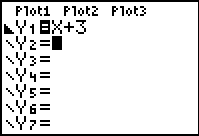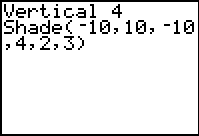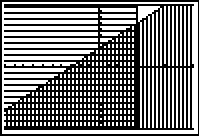You can shade the area between two graphs.  The Shade command shades the area that is specifically above the lower function and below the upper function.
Only the areas where lowerfunc < upperfunc are shaded.

Demo:  Solve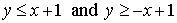• Enter Y1 = x + 1 and Y2 = -x + 1
• Graph to determine which graph is the "upperfunc".  In this problem it is Y1.  Think of the "upperfunc" as "function to shade below"(Be careful not to confuse the "upper" function with the "greater than" symbol.)
• Press DRAW (2nd PRGM) and choose option #7 Shade(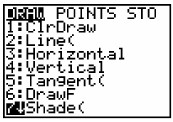Shade(function to shade above, function to shade below, left bound, right bound, the pattern (a number from 1 to 4), pattern resolution (a number from 1 to 8))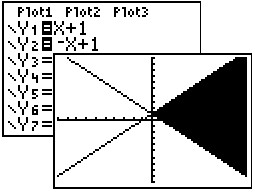Can I make it shade ONLY between three lines?

You have to be rather creative to get the calculator to shade only between three lines.
In most cases, it will be more work than worth.

Demo:  Solve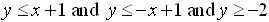Method 1:  (All shaded)Enter the inequalities into Y1, Y2 and Y3 and choose the proper "shade above" or "shade below" built-in icons in front of the Y=.  As you can see at the right, all areas are shaded and the answer is the darkest shading.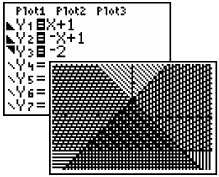Method 2:  (Answer only, shaded)Enter the inequalities without the built-in "shade above/below" icons.  This method will use the Boolean operators. Look carefully at the Y3 entry.  If the statement is true, a value of 1 is being multiplied times the function, and if false a value of 0.  The * is needed so that the function is not calculated as a composition of functions.  Then Shade (Y3, Y4) to get the solid figure.  Whew!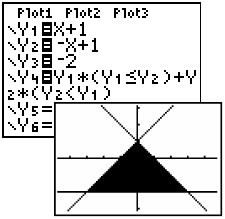Shade (Y3, Y4) to see graph.

 Demo:   Graph the solution set to this system of                  inequalities: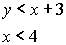Graph the first equation by entering into Y1 and choosing the "shade below" built-in icon. Choose DRAW (2nd PRGM) and #4 Vertical.  When the word Vertical appears on the home screen, type in your desired x-value.  In this case, you should have:   Vertical 4    (this will draw the vertical line x = 4) Choose DRAW (2nd PRGM) and #7 Shade. Type:  Shade( -10, 10, -10, 4, 2, 3) and hit Enter.  The Shade parameters are: -- the first two numbers are the range of y values you want to see -- the second two numbers are the range of x-values -- the fifth number is the pattern set to horizontal (default pattern is vertical) -- and the last number 3 will plot every 3rd pixel. The answer is the overlapped shading.